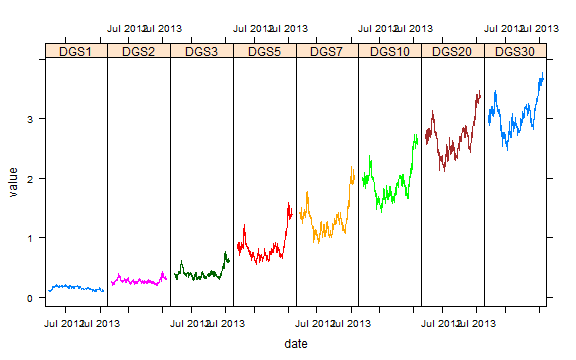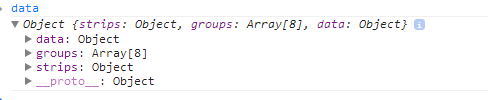# Multiple Lines gridSVG and d3As another post in the series, I thought I would show an alternate way of using d3 to bind data from a gridSVG exported lattice plot. This time we will have multiple strips complicating our data bind. Also, I will use a different set of techniques to send the data from R to Javascript. I think I prefer how I have done it previous posts though. No sense in wasting time with the intro. Let's grab US Treasury Yield Curve data from the Federal Reserve Bank of St. Louis FRED.

### FRED, Give Us Some Treasury Yield Data

We will get the daily yield series for US Treasuries with 1, 2, 3, 5, 7, 10, 20, and 30 year maturities.

``````require(quantmod)
require(rCharts)
#now get the US bonds from FRED
USbondssymbols <- paste0("DGS",c(1,2,3,5,7,10,20,30))

ust.xts <- xts()
for (i in 1:length( USbondssymbols ) ) {
ust.xts <- merge(
ust.xts,
getSymbols(
USbondssymbols[i], auto.assign = FALSE,src = "FRED"
)
)
}
ust.xts <- na.omit(ust.xts)

xtsMelt <- function(data) {
require(reshape2)

#translate xts to time series to json with date and data
#for this behavior will be more generic than the original
#data will not be transformed, so template.rmd will be changed to reflect

#convert to data frame
data.df <- data.frame(
cbind(format(index(data),"%Y-%m-%d"),coredata(data))
)
colnames(data.df) = "date"
data.melt <- melt(data.df,id.vars=1,stringsAsFactors=FALSE)
colnames(data.melt) <- c("date","indexname","value")
#remove periods from indexnames to prevent javascript confusion
#these . usually come from spaces in the colnames when melted
data.melt[,"indexname"] <- apply(
matrix(data.melt[,"indexname"]),
2,gsub,pattern="[.]",replacement=""
)
return(data.melt)
#return(df2json(na.omit(data.melt)))
}

ust.melt <- xtsMelt( ust.xts["2012::",] )

ust.melt\$date <- as.Date(ust.melt\$date)
ust.melt\$value <- as.numeric(ust.melt\$value)
ust.melt\$indexname <- factor(
ust.melt\$indexname, levels = colnames(ust.xts)
)
ust.melt\$maturity <- as.numeric(
substr(
ust.melt\$indexname, 4, length( ust.melt\$indexname ) - 4
)
)
ust.melt\$country <- rep( "US", nrow( ust.melt ))
``````

### Draw A Graph with Lattice

This graph could be much prettier, but to limit the amount of code I will settle for this uglier version.

``````require(latticeExtra)

p1 <- xyplot(
value ~ date | indexname,
groups = indexname,
data =  ust.melt,
type = "l",
scales = list(
x = list(
at = pretty(ust.melt\$date,n=3)[c(1,3)],
format = "%b %Y"
)),
layout = c(8,1)
)
p1
``````We will exploit `grid.export()` magic to export our graph to SVG.

``````p1
require(rjson)
require(gridSVG)

#export our lattice chart
exportlist <- grid.export("", addClasses = TRUE )
``````

### d3 Reverse Data Bind

Even though I don't like it as much, here is my original solution to sending data from R to javascript. You will probably notice that it requires much more R and javascript code to tidy and bind. This will result in data that looks something like this.``````#set up a function to pull the groups
#which lattice calls subscripts
#use with lapply
#l is the
getgroup <- function(l,p) {
return(p\$panel.args.common\$groups[l\$subscripts])
}

#set up a function to get the data and add groups
#in one list so we can pass as json
#accepts a trellis object as p
getlist <- function(p) {
data <- p\$panel.args

#use the getgroup function to get groups
#lattice places the groups in subscripts
#in the panel.args.common list
groups <- lapply(
data,
FUN=getgroup,
p=p
)

for ( i in 1:length(data) ) {
data[[i]]\$groups <- groups[[i]]
}

names(data) <- unlist(p\$condlevels)

return(
list(
strips = p\$condlevels,
groups = unique(unlist(groups)),
data = data
)
)
}

#use our fancy function from above to get
#all the data we will need to reverse data bind with d3
data <- rjson::toJSON( getlist( p1 ) )
cat('
<script>
data =
', data)
``````

The d3 reverse data bind portion of the javascript will look like this. We will loop through each of the keys (strips in lattice-speak) in the data. With each key, it will parse the points from the R/gridSVG line `pointsToArray(d3.select(g[i]).select('polyline').attr("points"))`. We will add `x,y,group, and strip` to our new `pointsdata` array.

``````var pointsdata = [];
var pointsline = [];
d3.entries(data.data).forEach(
function (d,i) {
pointsline = pointsToArray(d3.select(g[i]).select('polyline').attr("points"));
d.value.groups.forEach(
function (dd, ii) {
pointsdata.push(
{
x:pointsline[ii].x, y:pointsline[ii].y,
data: {"x": d.value.x[ii],
"y": d.value.y[ii],
"group": d.value.groups[ii],
"strip": d.key }
}
)
}
)
}
)

//assign data to the line
g.data(d3.nest().key(function (d) {
return d.data.group
}).entries(pointsdata));
``````

Once we have the data in bindable form and the points parsed, we can draw a path with d3. For now, I will make the stroke white, so that we can see our added paths.

``````//loop through each polyline and add a path to contain the data for tootips/hover
g.forEach(function(d) {
var mypath = d3.select(d).append("path")
mypath
.datum(mypath.datum().values)
.attr("d",line)
.attr("stroke","white")
})
``````

As usual, we can do lots of things with bound data. We'll build a little on R/gridSVG/d3 Line Reverse Data Bind by adding a similar mouseover, but this time for each of the lines. I also wanted to test if adding the yield curve would be helpful. Of course, this is a rough sketch and could be improved greatly by some simple styling. I'll leave that to you.